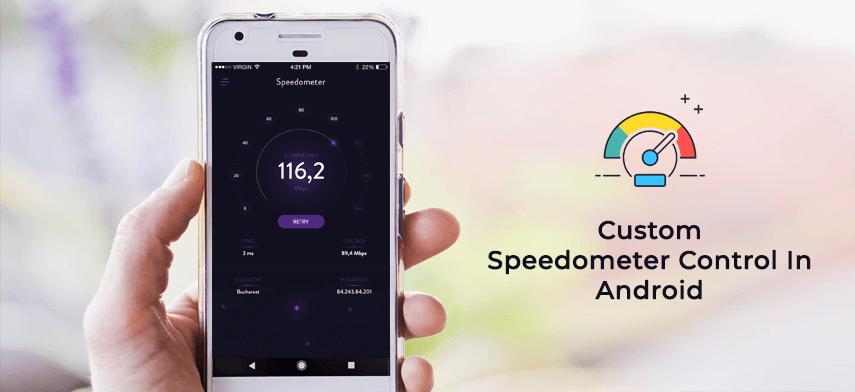We are going to draw and move the needle of custom speedometer using one of the unique and best feature of android for drawing that is Canvas and its on Draw method.We perform the moving animation of the needle by drawing it pixel by pixel on the canvas.

Lets start first by initializing all the drawables needed to draw the speedometer gauge and its needle.

For that lets create one view named SpeedoMeterView and have the following variables.

```Bitmap needle = null; //needle image
Bitmap speedo_meter = null;//main speedometer gauge.
Bitmap center_wheel = null;//center wheel.
```

We are having the images in drawables and for getting those images from drawable we have one function as

```public Bitmap getImage(int res_id) {
return BitmapFactory.decodeResource(getResources(), res_id);
}
```

So using this function we get the bitmaps as

```speedo_meter = getImage(R.drawable.meter);
center_wheel = getImage(R.drawable.center_wheel);
```

Some more variables and constants are;

```public static float minAngle = (float) 246.5;
public static float maxAngle = (float) 110.5;
```

minAngle and maxAngle values are set by Add-Hoc process.

```public static float exact_angle = 0;
```

exact_angle is the actual value on the speedometer gauge to which needle points.

```public static float angle_of_deviation = minAngle;
```

angle_of_deviation is the value on the screen which maps to exact_angle on the speedometer gauge.

```public static float maxValue = 100;
```

As we have largest value on the meter gauge 100; maxValue is set to 100.

Now the main function which calculates the angle of deviation based on the exact value passed to the function.

Here is that function

```public void calculateAngleOfDeviation(int randomly_generated_value) {

exact_angle = (float) randomly_generated_value;

float angleDef = (float) (maxAngle + minAngle - 130);

angle_of_deviation = ((exact_angle * angleDef) / maxValue) + minAngle;

speedo_obj.invalidate();
}
```

This is the main function which calculates the angle_of_deviation.

We pass the speed value to the calculateAngleOfDeviation function to which we want the needle to point that is the exact_angle.

Then calculated the angle difference and next the angle_of_deviation by using the formulae.

angle_of_deviation is the actual value on screen which maps the exact angle that is speed value.

Next important thing is that we call the onDraw method of canvas using

```speedo_obj.invalidate();
```

The invalidate() actually cause the onDraw method to execute.

OnDraw method performs all the draw function.

This includes

```canvas.drawBitmap(speedo_meter, (canvas.getWidth() / 2)- speedo_meter.getWidth() / 2,(canvas.getHeight() / 3)- speedo_meter.getHeight() / 2, null);

Matrix matrix_needle = new Matrix();

matrix_needle.setTranslate((canvas.getWidth() / 2)- needle.getWidth() / 2, (canvas.getHeight() / 3)- needle.getHeight());

matrix_needle.postRotate(angle_of_deviation, canvas.getWidth() / 2, 2 * needle.getHeight() - 10);

canvas.drawBitmap(needle, matrix_needle, paint_needle);

canvas.drawBitmap(center_wheel,(canvas.getWidth() / 2)- center_wheel.getWidth() / 2, (can	vas.getHeight() / 3)- center_wheel.getHeight() / 2, null);
```

Firstly we drawn the main Speedometer gauge and then the needle and finally the center wheel of the speedometer as shown in the screenshot.

As we are drawing the bitmaps we use canvas.drawBitmap() function of the canvas
to which we pass the bitmap which we want to draw and the respective x and y values where we wants these bitmap to draw.

For drawing needle used Matrix and its setTranslate and postRotate method which performs the translation and rotation animation on the needle and then drawn it on the canvas.

For drawing needle used one paint object paint_needle whose style property is set to Paint.Style.FILL and for sharpness its setAntiAlias property is set to true

Now we add this created view to the xml named speedo_meter as follows.

Now lets have one activity named speedoMeterActivity which will initialize this created view.

Initialize this view as

```SpeedoMeterView mView = (SpeedometerView) findViewById(R.id.speedometer_view);
```

We get the values for exact angle using the generateValue() as

```private void generateValue() {

Random r = new Random();

speedValueStr = String.valueOf(r.nextInt(100 - 10) + 10);

speedPreference.setPreviousNeedleValue(speedValueStr);

}
```

here we are generating the random values between 10 to 100 and storing temporarily in the preference for further use.

For demo purpose we are generating the value here;but it might happen the value may come through api.

For smooth rotation we will use thread and handler which gives calls to calculateAngleOfDeviation() function which in turn calls the onDraw method.

For this, defined one function moveNeedle() as,

```private void moveNeedle() {
handler = new Handler() {
public void handleMessage(android.os.Message msg) {

Bundle b = msg.getData();
int key = b.getInt(&quot;angle_in_degrees&quot;, 0);

if (key == 0) {

} else {
mView.calculateAngleOfDeviation(key);
}

};
};
handler.postDelayed(null, delay);

@Override
public void run() {

startAngle = Integer.parseInt(speedPreference.getPreviousNeedleValue());

generateValue();

if (Integer.parseInt(speedValueStr) &gt; 100) {
speedValueStr = &quot;100&quot;;
}
if (startAngle &gt; Integer.parseInt(speedValueStr)) {
for (int i = startAngle; i &gt;= Integer.parseInt(speedValueStr); i = i - 1) {
try {
Message msg = new Message();
Bundle b = new Bundle();
b.putInt(&quot;angle_in_degrees&quot;, i);
msg.setData(b);
handler.sendMessage(msg);
} catch (InterruptedException e) {

e.printStackTrace();
}
}

} else {
for (int i = startAngle; i &lt;= Integer.parseInt(speedValueStr); i = i + 1) {
try {

Message msg = new Message();
Bundle b = new Bundle();
b.putInt(&quot;angle_in_degrees&quot;, i);
msg.setData(b);
handler.sendMessage(msg);

} catch (InterruptedException e) {
e.printStackTrace();
}
}
}
}
});
}
```

In the run method we get the exact value from the preference and assign it to startAngle variable.

Initially startAngle will have value 0 as in the onCreate we are storing previous needle value in the preference to 0.

Now to move the needle forward and backward direction based on the previous needle value; for this we used to do one check as,

if (startAngle &gt; Integer.parseInt(speedValueStr))

So if the startAngle is greater than the current speedValue then we move needle in the backward direction by decreasing the value of i in the for loop else vice a versa and pass it to the handler which calls calulateAngleOfDeviation() and pass the value of i to it.

Thats it.